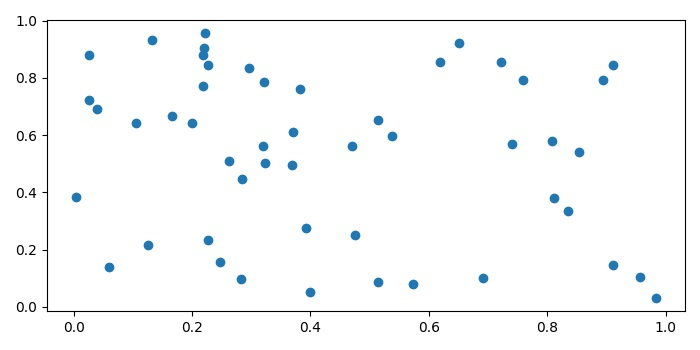# How I can get a Cartesian coordinate system in Matplotlib?

To plot a Cartesian coordinate system in matplotlib, we can take the following Steps −

• Initialize a variable (N) with a value.
• Create random data points for x and y.
• Plot the points using scatter method with x and y data points.
• To display the figure, use show() method.

## Example

import numpy as np
import matplotlib.pyplot as plt
plt.rcParams["figure.figsize"] = [7.00, 3.50]
plt.rcParams["figure.autolayout"] = True
N = 50
x = np.random.rand(N)
y = np.random.rand(N)
plt.scatter(x, y)
plt.show()

## Output